Next: Derivation of Radial Equation Up: Central Potentials Previous: Central Potentials

# Introduction

In this chapter, we shall investigate the interaction of a non-relativistic particle of massand energywith various so-called central potentials,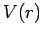, where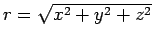is the radial distance from the origin. It is, of course, most convenient to work in spherical polar coordinates--,,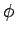--during such an investigation (see Sect. 8.3). Thus, we shall be searching for stationary wavefunctions,, which satisfy the time-independent Schrödinger equation (see Sect. 4.12)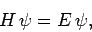(622)

where the Hamiltonian takes the standard non-relativistic form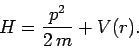(623)

Richard Fitzpatrick 2010-07-20